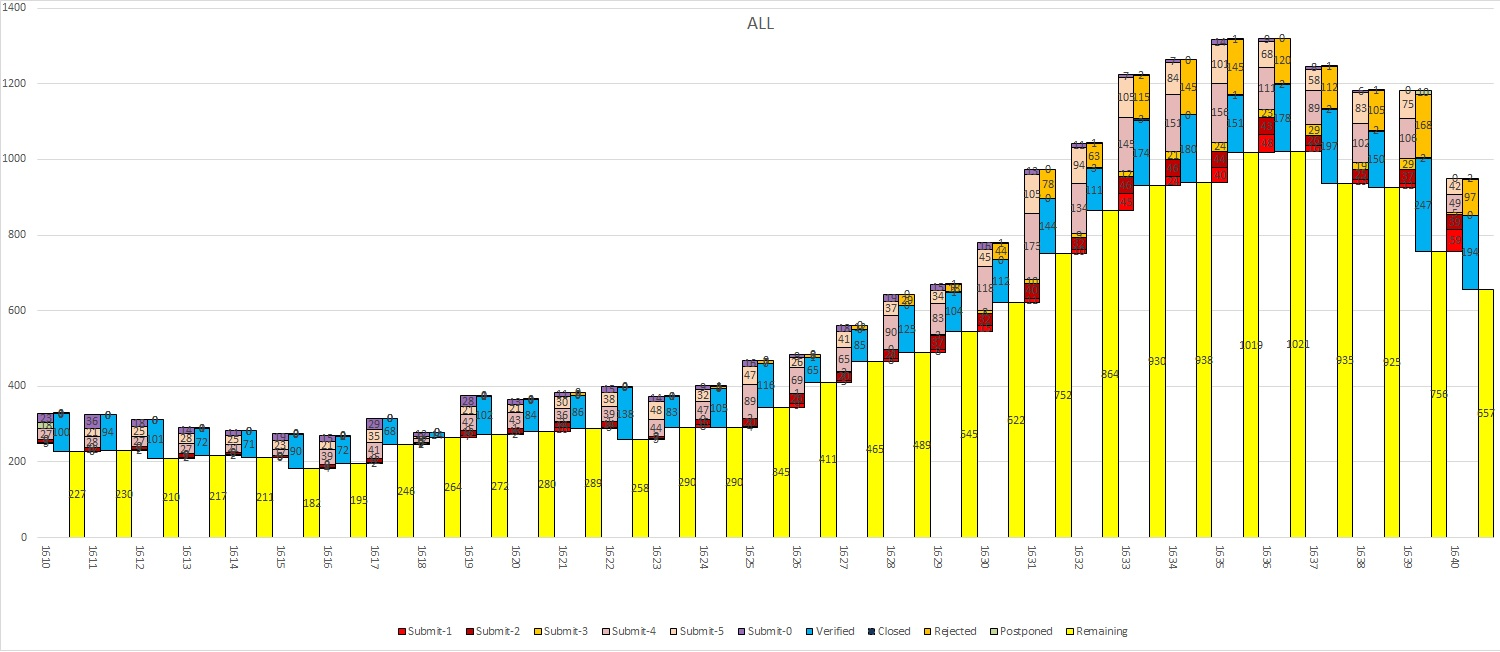# Qlik Deployment Framework

Announcements
BI & Data Trends 2021. Discover the top 10 trends emerging in today. Join us on Dec. 8th REGISTER
cancel
Showing results for
Did you mean:
HighlightedContributor III

## Stacked Bar chart ignore 2nd dimension

Hi

I wanted to produce a chart which can ignore 2nd dimension here 'Flag'. I am able to produce the graph using Excel but in need to get the same as interactive chart in QlikView.

Here is the data i prepared in Excel ChartData-1And i am able to produce below chart in Excelto create the same chart i have done back end processing in QlikView load script to format the data as ChartData-1 but this will be static and this data will not change based on selection in list box.

I was able to make the same data format in Pivot table, for that I created "Flag" Field to separate the data, but I am unable to get "Remaining" from it. since Remaining will be calculated based on Dimension, now i need to ignore the 2nd dimension in chart to get the exact value.

Here is the Pivot table which i created in QlikView.Where TotalSubmited= (Submit-1+Submit-2+Submit-3+Submit-4+Submit-0)

and Remaining = TotalSubmited - (Verified+Postponed+Closed+Rejected)

I need TotalSubmited and Remaining to have same value for both the flag in a week.

Pleas help solving this problem. Thanks in advance.

1 Solution

Accepted Solutions
HighlightedContributor III

Hi All,

I found the solution by using below expression.

Alt(Above(TOTAL if(Flag=1,  RealTotalSubmit)))

Thanks for trying.

Regards

Purushotham

6 Replies
HighlightedMVP & Luminary

Can you post the excel file?

talk is cheap, supply exceeds demand
HighlightedMVP

What are the expressions behind Submit-1, Submit-2, Submit-3 etc? Making an assumption that they are Sum(Measure1), Sum(Measure2), Sum(Measure3) and so on, this is what you can try:

Total Submitted

RangeSum(

Sum(TOTAL <Week> Measrure1),

Sum(TOTAL <Week> Measrure2),

Sum(TOTAL <Week> Measrure3),

Sum(TOTAL <Week> Measrure4),

Sum(TOTAL <Week> Measrure0))

Remaining

[Total Submitted] -

RangeSum(

Sum(TOTAL <Week> Verified),

Sum(TOTAL <Week> Postponed),

Sum(TOTAL <Week> Closed),

Sum(TOTAL <Week> Rejected))

So the main thing here is the use of TOTAL here. Read about it here: What does the TOTAL qualifier do?

HighlightedContributor III

Hi,

Here is the excel containing example. please do the needful.

HighlightedMVP & Luminary

Use this script:

CrossTable(Status, Value,2)

Floor(Flag) as Flag,

[Submit-1],

[Submit-2],

[Submit-3],

[Submit-4],

[Submit-5],

[Submit-0],

Verified,

Closed,

Rejected,

Postponed,

Base1,

Base2,

Base3

FROM

[ChartData.xlsx]

(ooxml, embedded labels, table is ALL_D, filters( Replace(1, top, StrCnd(null))))

;

Then create a bar chart with the following dimensions:

• wk
• Flag
• Status

And use sum(Value) as expression.

Click on the + in front of the expression on the Expressions tab, select Background color and enter an expression that sets the background color for Base1 and Base2 to transparent: if(Match(Status, 'Base1','Base2'),red(0),color(FieldIndex('Status',Status)))

talk is cheap, supply exceeds demand
HighlightedContributor III

Hi Thanks for the response.

I have huge data to be processed behind and I can't include that data as sample.

but I am able to get exact values as chart below, but i am unable to get the previous row value using

Here is the formula

RealTotalSubmit= (Submit-1 + Submit-2 + Submit-3 + Submit-4 + Submit-0)

RealToalVarified= (Verified+Postponed+Closed+Rejected)

Base1 =if(Flag=1,  RealTotalSubmit)

Base2 =if(Flag=1,  RealTotalSubmit)

Base3 =if(Flag=1,  RealTotalSubmit)

I tried 'Base1 = Above(Base3)', but not working.

I can get accumulation in bar chart so in just need Base1 to get the previous Remaining value for Base3 or Base2HighlightedContributor III

Hi All,

I found the solution by using below expression.

Alt(Above(TOTAL if(Flag=1,  RealTotalSubmit)))

Thanks for trying.

Regards

Purushotham